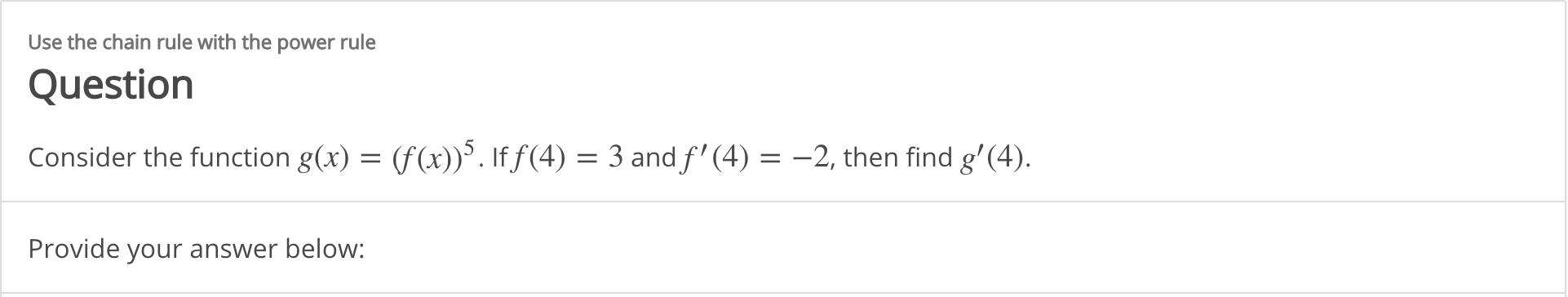# Use the chain rule with the power ruleQuestionConsider the function g(x) = (f(x)). If f(4) = 3 and f' (4) = -2, then find g'(4).Provide your answer below:

Question
2 viewshelp_outlineImage TranscriptioncloseUse the chain rule with the power rule Question Consider the function g(x) = (f(x)). If f(4) = 3 and f' (4) = -2, then find g'(4). Provide your answer below: fullscreen
check_circle

star
star
star
star
star
1 Rating
Step 1

Given function:

Step 2

Apply the chai...

### Want to see the full answer?

See Solution

#### Want to see this answer and more?

Solutions are written by subject experts who are available 24/7. Questions are typically answered within 1 hour.*

See Solution
*Response times may vary by subject and question.
Tagged in

### Other